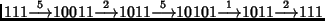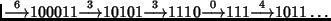## Siteswap

A siteswap is a sequence encountered in Juggling in which each term is a Positive integer, encoded in Binary. The transition rule from one term to the next consists of changing some 0 to 1, subtracting 1, and then dividing by 2, with the constraint that the Division by two must be exact. Therefore, if a term is Even, the bit to be changed must be the units bit. In siteswaps, the number of 1-bits is a constant.

Each transition is characterized by the bit position of the toggled bit (denoted here by the numeral on top of the arrow). For example,The second term is given from the first as follows: 000111 with bit 5 flipped becomes 100111, or 39. Subtract 1 to obtain 38 and divide by two to obtain 19, which is 10011.